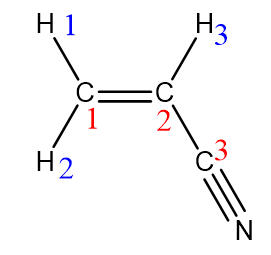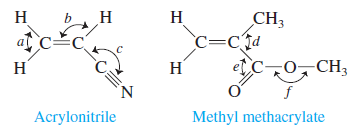# Problem: Many important compounds in the chemical industry are derivatives of ethylene (C2H4). Two of them are acrylonitrile and methyl methacrylate.Complete the Lewis structures, showing all lone pairs. Give approximate values for bond angles a through f. Give the hybridization of all carbon atoms. In acrylonitrile, how many of the atoms in the molecule must lie in the same plane? How many σ bonds and how many π bonds are there in methyl methacrylate and acrylonitrile?

###### FREE Expert Solution

The steps that we are required to take in order to do this problem are as follows:

Step 1: Placing the lone pairs of electrons

Acrylonitrile

• There are 20 valence electrons present. You will then need to count the number of bonds present and subtract two electrons for each bond.

Total Number of bonds = 9

Electrons: 9 x 2 = 18 e90% (429 ratings)###### Problem Details

Many important compounds in the chemical industry are derivatives of ethylene (C2H4). Two of them are acrylonitrile and methyl methacrylate.Complete the Lewis structures, showing all lone pairs. Give approximate values for bond angles a through f. Give the hybridization of all carbon atoms. In acrylonitrile, how many of the atoms in the molecule must lie in the same plane? How many σ bonds and how many π bonds are there in methyl methacrylate and acrylonitrile?